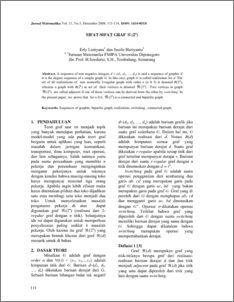# SIFAT-SIFAT GRAF (2n)

Listiyana, Erly and Hariyanto, Susilo (2008) SIFAT-SIFAT GRAF (2n). JURNAL MATEMATIKA MATEMATIKA, 11 (3). pp. 111-114. ISSN 1410-8518Preview
PDF
147Kb

## Abstract

A sequence of non negative integers d = (d1, d2, …, dn) is said a sequence of graphic if it is the degree sequence of a simple graph G. In this case, graph G is called realization for d. The set of all realizations of non isomorfic 2-regular graph with order n (n ≥ 3) is denoted R(2n), whereas a graph with R(2n) as set of their vertices is denoted (2n) . Two vertices in graph (2n) are called adjacent if one of these vertices can be derived from the other by switching. In the present paper, we prove that for n ≥ 6, (2n) is a connected and bipartite graph.

Item Type: Article Sequences of graphic, bipartite graph, realization, switching , connected graph. Q Science > QA Mathematics Faculty of Science and Mathematics > Department of Mathematics 1953 INVALID USER 01 Dec 2009 01:39 04 Dec 2009 09:04

Repository Staff Only: item control page